# Inverse Properties of Addition and Multiplication

Inverse properties âundoâ each other. The purpose of the inverse property of addition is to get a result of zero. The purpose of the inverse property of multiplication is to get a result of 1. We use inverse properties to solve equations.

Inverse Property of Addition says that any number added to its opposite will equal zero. What is the opposite you might ask? All you have to do is change the sign from positive to negative or negative to positive.

Let's see what that looks like.

Example 1:     5 + (-5) = 0     -5 is the opposite of 5

Example 2:     -4 + (4) = 0    -4 is the opposite of 4

Sometimes this can be written in a vertical format.

Example 3:        10

-10    -10 is the opposite of 10

0

Example 4:      -12

+12     12 is the opposite of - 12

0

Inverse Property of Multiplication says that any number multiplied by its reciprocal is equal to one.

Let's start by defining a reciprocal. To find the reciprocal of any number write it as a fraction and then flip it.

Example 1: find the reciprocal of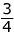.    Flip it →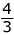.

The reciprocal of. is.

Example 2: find the reciprocal of 5. → Write it as a fraction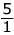→flip it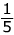The reciprocal of 5 isExample 3: find the reciprocal of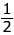.  → flip it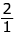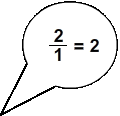The reciprocal ofis 2

Example 4: find the reciprocal of -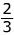. → flip it -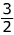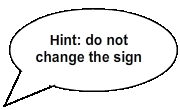The reciprocal of -is -Special Reminder: To multiply fractions you multiply the numerator times the numerator and then the denominator times the denominator and then simplify your answer: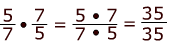= 1

Now let's look at how we can use this with the inverse of multiplication.

(number)(reciprocal) = 1

Example 1:= 1   →= 1

Example 2:  7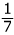= 1 →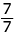= 1

Now let's summarize what we have learned.

The Inverse Property of Addition says that any number added to its opposite is equal to zero.

a + (-a) = 0

The Inverse Property of Multiplication says that any number multiplied by its reciprocal is equal to 1.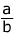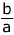= 1

Math
algebra
Trichotomy Property
Distributive Property

To link to this Inverse Properties of Addition and Multiplication page, copy the following code to your site: